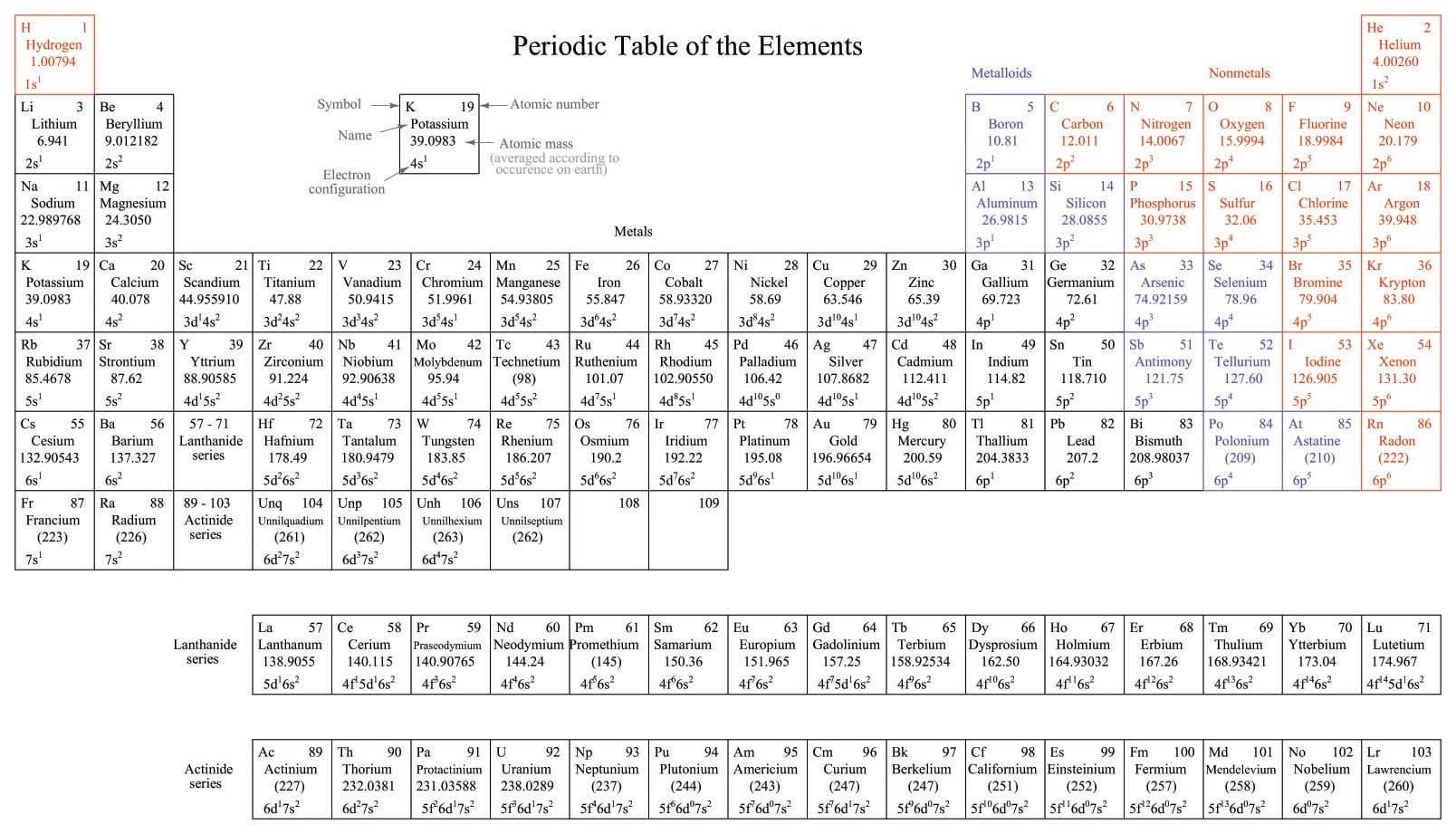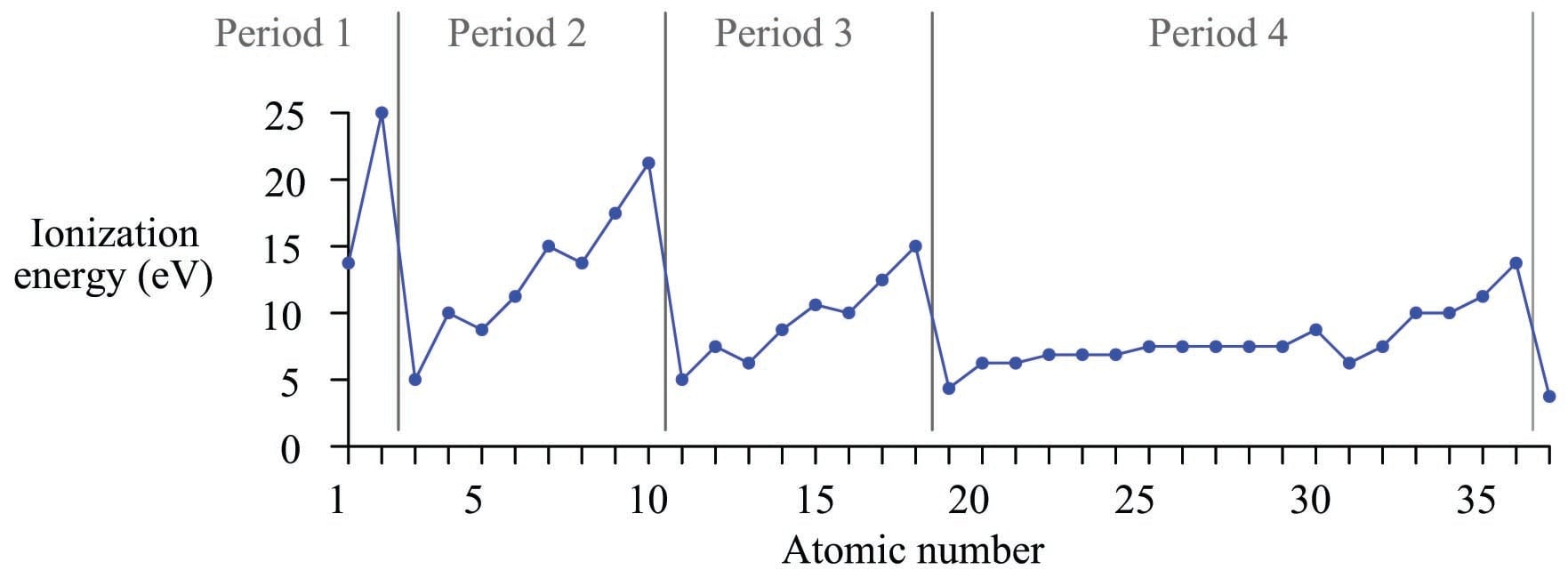Periodic Table of the Elements

Chapter 3 - Chemistry in Industrial Instrumentation

All substances are comprised of various elements in various combinations and proportions. Elements may thus be thought of as the building-blocks of matter. A Periodic Table of the Elements is a table listing the known elements in order of their atomic numbers.Multiple attributes appear for each element in the table. Two of these attributes – atomic number and atomic mass – are directly related to the number of particles in the nucleus of each atom. We will examine the table’s entry for the element potassium (K) to explore these concepts.

Potassium has an atomic number (number of protons in the nucleus of each potassium atom) of 19. This number defines the element. If we were somehow to add or subtract protons from the nucleus of a potassium atom, it would cease being potassium and transmutate into a different element. Note how every element in the table has its own unique atomic number, and how each of these numbers is whole (no fractions or decimals).

The atomic mass or atomic weight shown for potassium is 39.0983. This quantity is the sum of protons and neutrons found in the nucleus of each potassium atom. Like the atomic number (19), we would logically expect the atomic mass to be a whole number as well, since protons and neutrons only come in whole quantities. The primary reason we see a non-whole number for potassium’s atomic mass is that this table reflects the average atomic mass of potassium atoms as found in nature. Some potassium atoms have atomic masses greater than 39, and some have atomic masses less than 39. We know that the number of protons in every potassium atom is fixed (which is what gives potassium its elemental identity), which means the only quantity that may cause the atomic mass to vary is the number of neutrons in the nucleus. The most common form of potassium ($$^{39}$$K) atom possesses 19 protons and 20 neutrons in its nucleus, giving it an atomic mass of 39 (19 + 20). The next most common form of potassium found on Earth is ($$^{41}$$K), possessing 19 protons and 22 neutrons.

We refer to atoms of the same element with differing atomic masses as isotopes. From a chemical perspective, isotopes are identical. That is to say, they engage in the exact same chemical reactions in the exact same manner. To use potassium as an example, an atom of $$^{39}$$K will join with a chlorine atom (Cl) to form the compound potassium chloride (KCl) just as readily as an atom of $$^{41}$$K will join with a chlorine atom to form the same compound. The three isotopes of hydrogen ($$^{1}$$H, $$^{2}$$H, and $$^{3}$$H: hydrogen, deuterium, and tritium, respectively) are all chemically identical: all are highly flammable, combining with oxygen to create water (H$$_{2}$$O). However, deuterium ($$^{2}$$H) has twice the density of normal hydrogen ($$^{1}$$H), while tritium ($$^{3}$$H) has three times the density of normal hydrogen and is highly radioactive! Isotopes only differ in their mass and in their nuclear properties (such as radioactivity: the tendency for a nucleus to spontaneously decay, usually resulting in a loss or gain of protons that subsequently alters the identity of the decayed atom).

The Periodic Table is called “periodic” because its configuration reveals a repeating pattern of chemical behaviors approximately following atomic number. Horizontal rows in the table are called periods, while vertical columns are called groups. Elements in the same group (vertical column) share similar chemical reactivities – that is, they tend to engage in the same types of chemical reactions – despite having different masses and physical properties such as melting point, boiling point, etc. This periodicity is a function of how electrons are arranged around the nucleus of each atom, a subject we will explore in more detail later in this chapter. As mentioned previously, chemistry is the study of how atoms bond together to form molecules, and this bonding takes place through the interaction of the electrons surrounding the atoms’ nuclei. It makes perfect sense, then, that the configuration of those electrons determine the chemical (bonding) properties of atoms.

Some periodic tables show the first ionization energy value for each element – the amount of energy required to force the first electron of an electrically balanced atom to separate from that atom – in addition to other attributes such as atomic number and atomic mass. If we note the ionization energies of the elements, reading each element in turn from left-to-right, starting with period 1 (hydrogen and helium) and progressing to subsequent periods, we see an interesting pattern:

& & (measured in electron-volts'') \cr

Element Period First ionization energy Hydrogen (H) 1 13.5984 Helium (He) 1 24.5874 Lithium (Li) 2 5.3917 Beryllium (Be) 2 9.3227 Boron (B) 2 8.298 Carbon (C) 2 11.2603 Nitrogen (N) 2 14.5341 Oxygen (O) 2 13.6181 Fluorine (F) 2 17.4228 Neon (Ne) 2 21.5645 Sodium (Na) 3 5.1391 Magnesium (Mg) 3 7.6462 Aluminum (Al) 3 5.9858 Silicon (Si) 3 8.1517 Phosphorus (P) 3 10.4867 Sulfur (S) 3 10.36 Chlorine (Cl) 3 12.9676 Argon (Ar) 3 15.7596 Potassium (K) 4 4.3407

First ionization energy represents the relative stability of the last electron balancing the electrical charge of an atom. We see from this table that 24.5874 electron-volts of energy is needed to remove one electron from an electrically-balanced atom of helium (changing He into He$$^{1+}$$), while only 13.5984 electron-volts of energy is required to do the same to an atom of hydrogen. This tells us the electron configuration of helium is at a lower energy (and therefore more stable) than that of hydrogen.

The ionization energies increase with increasing atomic number (with an occasional down-step) until the last column of the period is reached, and then there is a comparatively enormous down-step in energy at the first column of a new period. This pattern is clearly evident when the first ionization energies are plotted against atomic number:This periodicity suggests that as atoms grow in atomic number, the additional electrons do not simply pile on in random fashion or in a plain and simple progression from inner orbits to outer orbits. Rather, they “fill in” a structured energy pattern, with major changes in structure at the start of each new period. More details of this structured pattern will be explored later in this chapter.

The low ionization energy values for all the “Group 1” elements (far left-hand column) suggest they are relatively easy to positively ionize, and indeed we find this to be the case through experimentation. Hydrogen, lithium, sodium, potassium, and the rest all readily become positively-charged ions upon interaction with other atoms, since their low ionization energy values means they may easily lose an electron.

The high ionization energy values for all the “Group 18” elements (far right-hand column) suggest they possess a very stable electron structure, which is also verified by experiment. These are the noble elements, possessing very little reactive potential.

Looking at the “Group 17” column, just to the left of the noble elements, we notice that they are all just one electron shy of the stable electron structure enjoyed by the noble atoms when in their electrically-balanced states. This suggests it might be easy to add one more electron to atoms of these elements, which (once again!) is a principle validated by experiment. Fluorine, chlorine, bromine, iodine, and even astatine all readily ionize negatively, readily accepting an extra electron from surrounding atoms. As one might expect from this tendency, these elements readily bond through electrostatic attraction with the “Group 1” elements (hydrogen, lithium, sodium, potassium, etc.), each “Group 17” atom accepting an extra electron from each “Group 1” atom which readily provides it. Ordinary table salt (sodium chloride, or NaCl) is an example of a compound formed by this sort of bond.

Thus, Group 1 and Group 17 elements are both highly reactive in a chemical sense, but in different ways. Group 1 elements easily form bonds with Group 17 elements, but Group 1 elements do not generally bond (solely) with other Group 1 elements, and likewise Group 17 elements do not generally bond (solely) with other Group 17 elements. It is the structure of the electrons surrounding each atom’s nucleus that determines how those atoms will bond with other atoms.

Published under the terms and conditions of the Creative Commons Attribution 4.0 International Public License